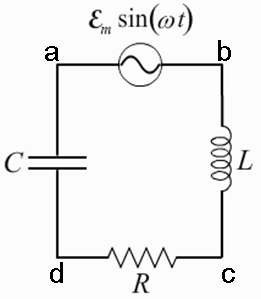# Problem: A circuit is constructed with an AC generator, a resistor, capacitor and inductor as shown. The generator voltage varies in time as ε =Va - Vb = εmsinωt, where εm = 120 V and ω = 737 radians/second. The values for the remaining circuit components are: R = 83 Ω, L = 112.5 mH, and C = 11.6μF.What is t1, the first time after t = 0 when the voltage across the inductor is zero?

###### FREE Expert Solution

Current:

The voltage across the inductor:###### Problem Details

A circuit is constructed with an AC generator, a resistor, capacitor and inductor as shown. The generator voltage varies in time as ε =Va - Vb = εmsinωt, where εm = 120 V and ω = 737 radians/second. The values for the remaining circuit components are: R = 83 Ω, L = 112.5 mH, and C = 11.6μF.What is t1, the first time after t = 0 when the voltage across the inductor is zero?